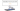# Assessable Value Calculation For Imports

By | July 9, 2020Hello, friends welcome to another fresh article of “IndianCustoms.Info”. Here in this article, I explained how to calculate Assessable value, IGST Value, customs duty and Landed value.

Once when I search on google to know the latest method to calculate AV. I did not find any updated calculation method.

After checking lots of sites, I came to know that all the sites providing an old method of calculating the assessable value.

Wasting lots of time on the internet I find that On 26th September 2017, the calculation method of AV was changed.

But still, none of the sites updates the new method of AV calculation. So, I decided to explain the new method of AV calculation on this blog.

I’m sure that this article will solve all your queries regarding the Assessable value, IGST Value, customs duty and Landed value calculation.

This article contains all the methods of calculating values covered under import customs clearance.

## How To Calculate Assessable Value?

If you want to know the AV calculation method in Hindi you can also watch the below video or continue the read article.

In the above video, I had explained how you can calculate the AV by using our Import Duty Calculator.

By using our Import duty calculator you can easily calculate the IGST Value, customs duty, Landed value, etc.

Let’s continue the article!

Mainly AV is calculated for the calculation of customs duty.

To calculate the AV, You need to calculate the CIF value.

As per Circular 39/2017-Customs, The CIF value and Assessable value are the same.

CIF (Cost, Insurance, Freight) value is the total value of “Invoice value + Insurance + Freight + Ex-work charges (If any)”.

For Example:-

• Invoice Value = Rs.1000/-
• Insurance Value = Rs. 11.25/-
• Freight Value = Rs.200/-

Rs.1000 (Invoice Value) + Rs. 11.25 (Insurance Value) + Rs.200 (Freight Value) = Rs.1211.25/- (CIF value)

CIF value = Rs.1211.25/- (Assessable Value)

Note:- The above calculation is for FOB & Ex-Work shipments.

## How To Calculate Customs Duty?

Customs duty is calculated on the basis of AV. Here below I had explained the complete calculation process.

Before start, you need to under what is the total customs duty and what included in total customs duty.

In normal import Customs duty is the total of BCS, SWS & IGST.

• BCD – Basic customs Duty
• SWS – Social Welfare Surcharge
• IGST – Integrated Goods and Services Tax

If BCD (Basic Duty) is 10%, SWS (Social welfare surcharge) is 10%, and IGST is 18%

BCD:- Rs.1211.25/- (AV) x 10% = RS.121.125/- (Basic Duty).

SWS:- Rs.121.125 (Basic Duty) x 10% = Rs.12.1125/- (Social welfare surcharge)

IGST:- [Rs.1211.25 (AV) + Rs.121.125 (BCD) + Rs.12.1125/- (SWS)] x 18% = Rs.242.00775/- (IGST Amount)

Total Customs duty (Excluding IGST amount) = Rs.133.2375/-

Total Customs duty (Including IGST amount) = Rs.375.24525/-

## How To Calculate IGST Assessable Value?

Igst assessable value calculation is very simple.

To calculate IGST AV you need to add Assessable Value + Basic Customs Duty + Social Welfare Charges.

For Example:-

IGST value Calculation :- Rs.1211.25 (AV) + Rs.121.125 (BCD) + Rs.12.1125/- (SWS) = Rs.1344.4875/- (IGST Value)

## How To Calculate Landed Value?

If you have already calculated the AV and BCD, then Landed value calculation very easy for you.

To calculate Landed value you need to Add Assessable value (AV) and basic customs duty (BCD).

For Example:-

Assessable value (AV) = Rs.1211.25/- + Basic Customs Duty (BCD) = RS.121.125/-

Landed Value = 1,332.375 (LV)

“I hope now you understand the new method of AV calculation for import customs duty in India. Comment below if anyone has any suggestions or queries regarding the Assessable Value, IGST Value, and Customs duty calculation.”

## 9 thoughts on “Assessable Value Calculation For Imports”

1.Bandarkiu Online

What is the scope of change in assessable value calculation in the future.

1.admin Post author

Assessable value calculation may change in the future but no guarantee for the same.

If there are any changes happen in the coming future I will update the same in this article.

Thanks & Regards
(IndianCustoms.Info)

2.Kiran kumar

Invoce amount in usd is 19528 dollar Invoice value cif inr is 1374771.20 freight is 44000 and insurance 1279 rs what is the assessable value please forward in mail

1.Admin (IndianCustoms.Info) Post author

Mr. Kiran Kumar,

CIF value means “Cost, Insurance, Freight“.
In this case, You already have CIF value,
CIF value is your assessable value.

3.naveen

WE HAVE IMPORTED SEVERAL CONSIGNMENTS AND IN ALL CASES THE FREIGHT ( ACTUAL ) WAS CONSIDERED FOR CALCULATION OF AV. THIS WAS ABOUT 12-14% OF COST. IN ONE CASE IT WAS TAKEN AS 20%FOR THE PURPOSE OF CALCULATING AV ( THIS WAS A COURIER SHIPMENT). .THE ACTUAL FREIGHT WAS 2%.. WHAT COULD BE THE REASONS FOR CONSIDERING THIS HIGH FREIGHT FOR CALCULATING AV

1.Admin (IndianCustoms.Info) Post author

Your clearing agent may use 20% of freight instead of the actual freight at the time of B.E filing.

4.Sambhav Jain

Dear Sir,
if i received a quotation in FOB value then how can i considered the value of ocean/air freight and insurance value.
Freight 20% of FOB value and insurance 1.125% is thumb rule or it is valid for calculate CIF value.

1.Admin (IndianCustoms.Info) Post author

If you get FOB value and want to calculate CIF value then you need to consider freight as 20% of FOB value and Insurance as 1.125% of FOB value.

1.Sambhav Jain